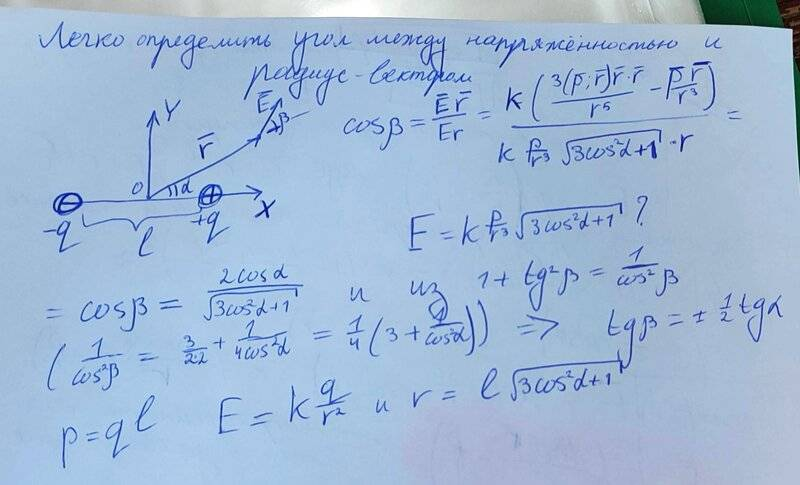# Dipole in polar coordinates

DaraRychenkova
Homework Statement:
- Determination of the dipole (p=ql). Find the dipole potential at a distance r much larger than the size of the dipole itself. Calculate the field of the dipole using the relationship between the potential and the field.

1. Solve the problem of finding the dipole field using the expression for the potential obtained in the previous problem in polar coordinates
Relevant Equations:
Dipole, electrostatic
I don't know how to get the result referring to the previous task. Is my decision correct?#### Attachments

Homework Helper
Gold Member
The potential in the "previous problem" is probably something like $$V=\frac{1}{4\pi\epsilon_0}\frac{\mathbf{p}\cdot\mathbf{r}}{r^3}.$$ If it is in some other form, use that. What do you think "the relationship between the potential and the field" is?

•MatinSAR, vanhees71 and PhDeezNutz
$$\vec{E}=\frac{1}{4 \pi \epsilon_0 r^5}(3 \vec{r} \vec{r} \cdot \vec{p}-r^2 \vec{p}).$$
•